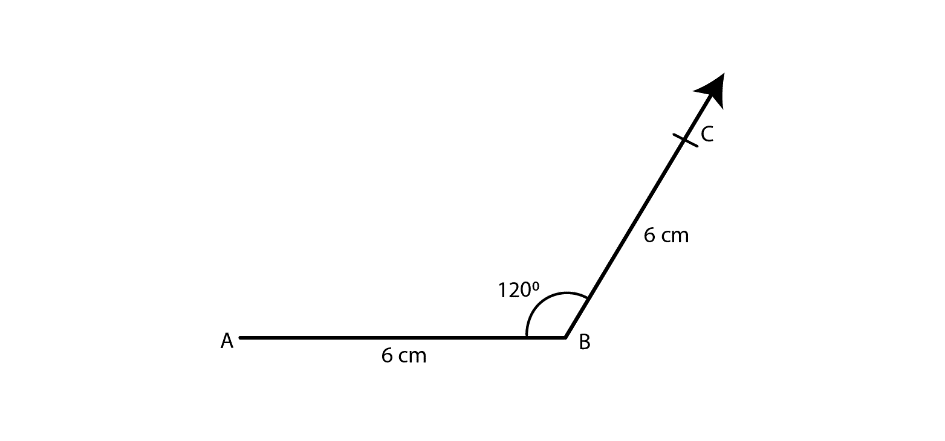# RD Sharma Solutions for Class 8 Maths Chapter 18 Practical Geometry (Constructions) Exercise 18.3

Construction of a quadrilateral when its four sides and one diagonal are given are explained in this Exercise 18.3, Chapter 18 of RD Sharma. Steps to construct a quadrilateral are explained in a step by step manner to help students easily understand the concept and can easily follow the steps for construction. One who practices regularly can gain command over the subject. Students can refer to RD Sharma Class 8 Solutions pdf and can download from the links given below and start practising offline.

## Download the Pdf of RD Sharma Solutions for Class 8 Maths Exercise 18.3 Chapter 18 Practical Geometry (Constructions)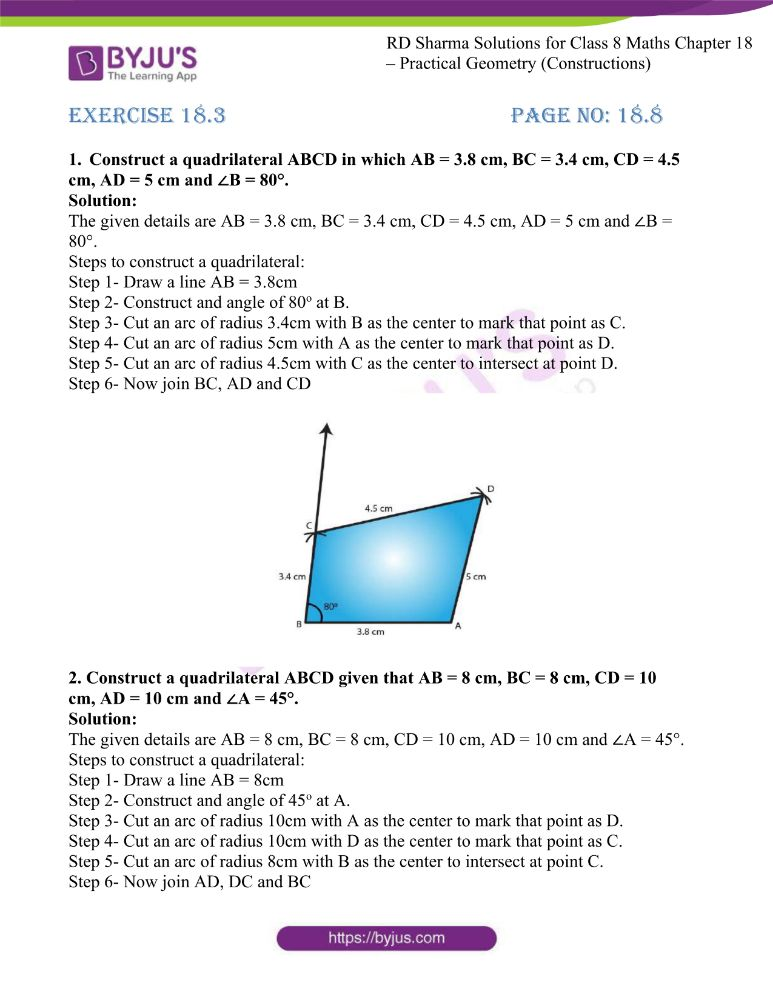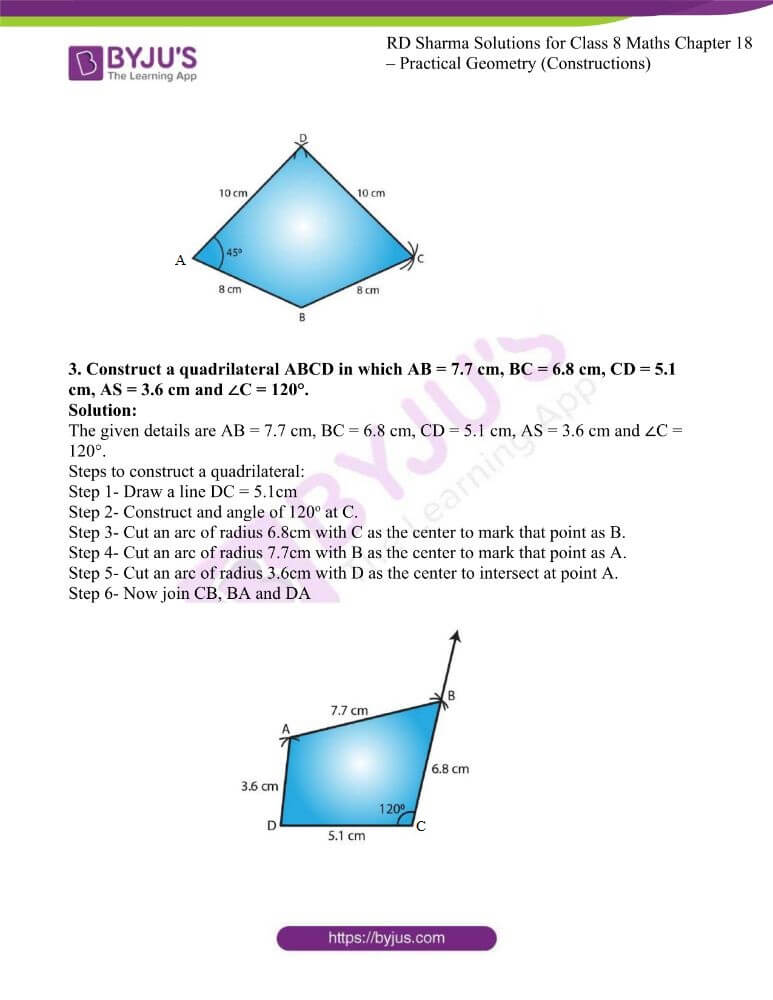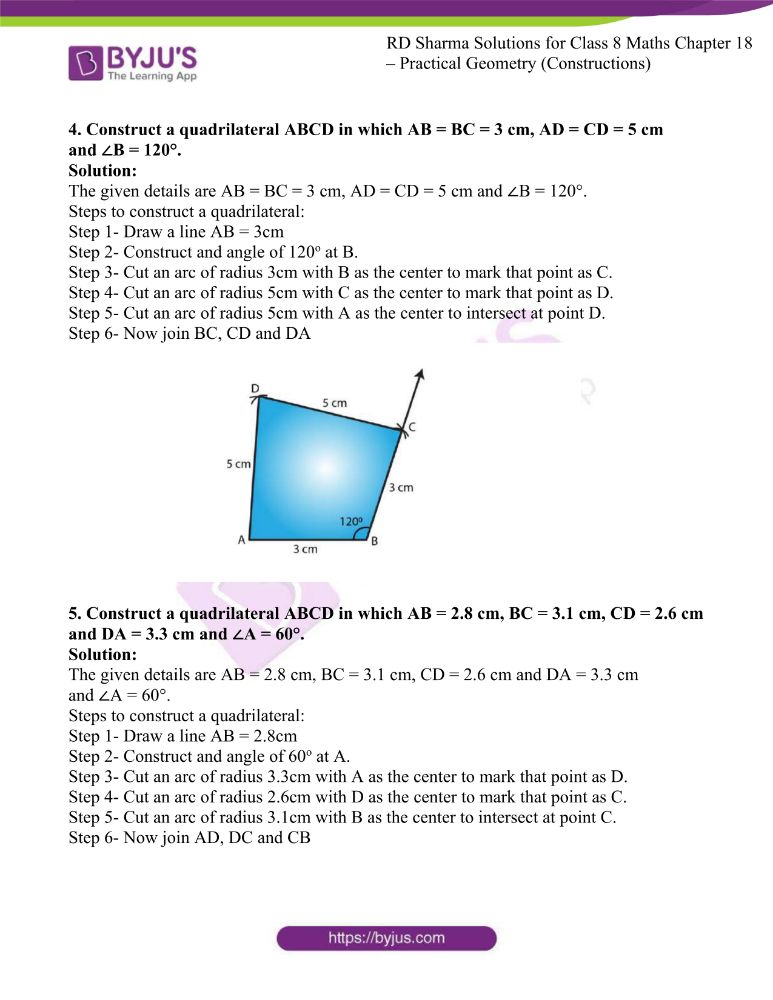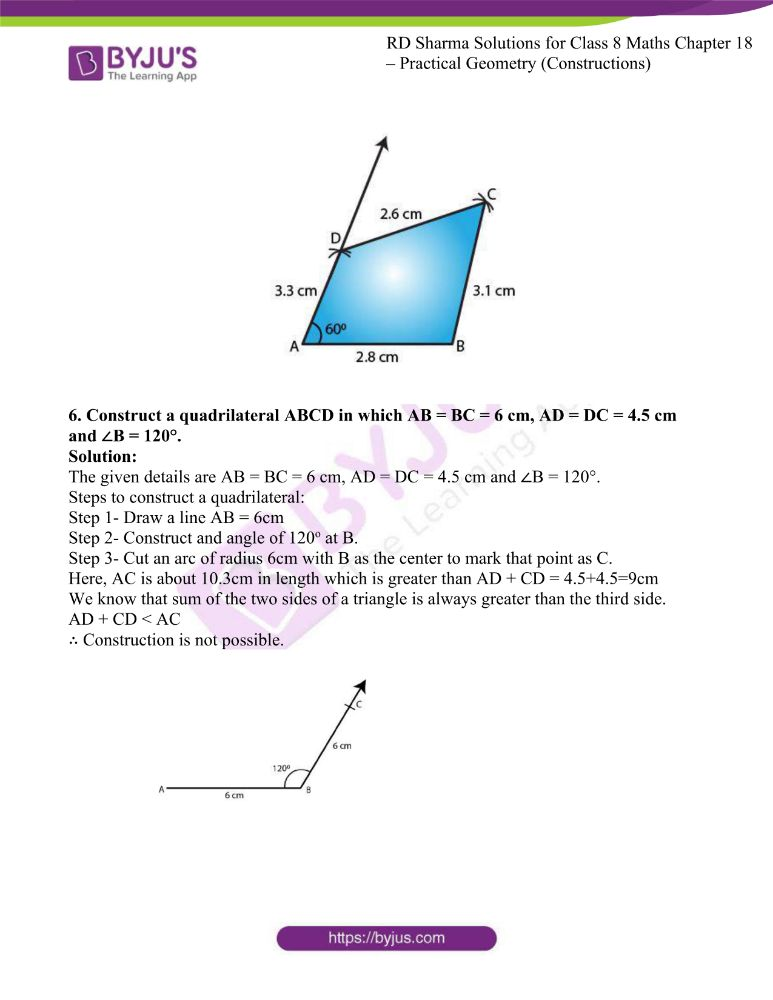### Access answers to RD Sharma Maths Solutions For Class 8 Exercise 18.3 Chapter 18 Practical Geometry (Constructions)

1. Construct a quadrilateral ABCD in which AB = 3.8 cm, BC = 3.4 cm, CD = 4.5 cm, AD = 5 cm and ∠B = 80°.

Solution:

The given details are AB = 3.8 cm, BC = 3.4 cm, CD = 4.5 cm, AD = 5 cm and ∠B = 80°.

Step 1- Draw a line AB = 3.8cm

Step 2- Construct and angle of 80o at B.

Step 3- Cut an arc of radius 3.4cm with B as the center to mark that point as C.

Step 4- Cut an arc of radius 5cm with A as the center to mark that point as D.

Step 5- Cut an arc of radius 4.5cm with C as the center to intersect at point D.

Step 6- Now join BC, AD and CD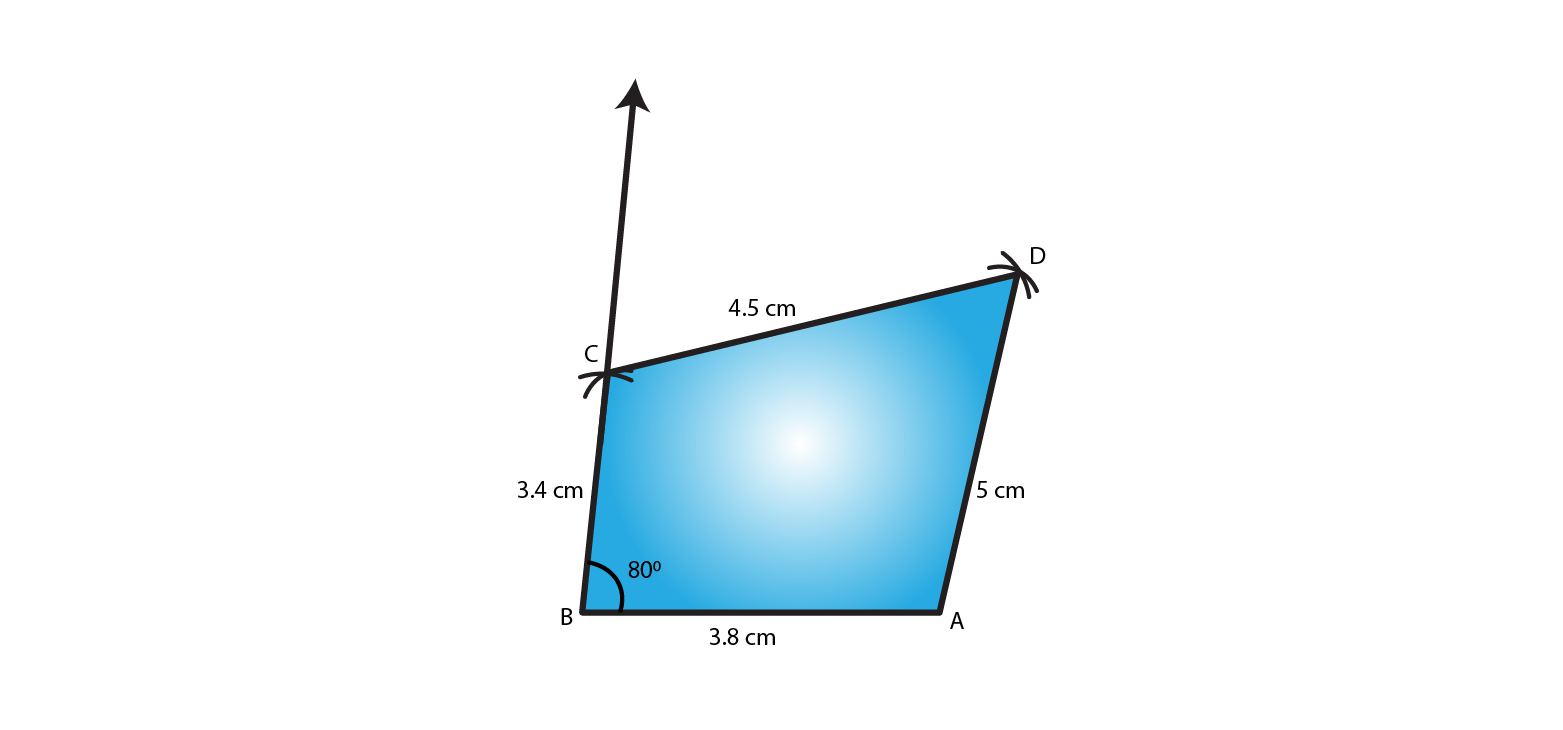2. Construct a quadrilateral ABCD given that AB = 8 cm, BC = 8 cm, CD = 10 cm, AD = 10 cm and ∠A = 45°.

Solution:

The given details are AB = 8 cm, BC = 8 cm, CD = 10 cm, AD = 10 cm and ∠A = 45°.

Step 1- Draw a line AB = 8cm

Step 2- Construct and angle of 45o at A.

Step 3- Cut an arc of radius 10cm with A as the center to mark that point as D.

Step 4- Cut an arc of radius 10cm with D as the center to mark that point as C.

Step 5- Cut an arc of radius 8cm with B as the center to intersect at point C.

Step 6- Now join AD, DC and BC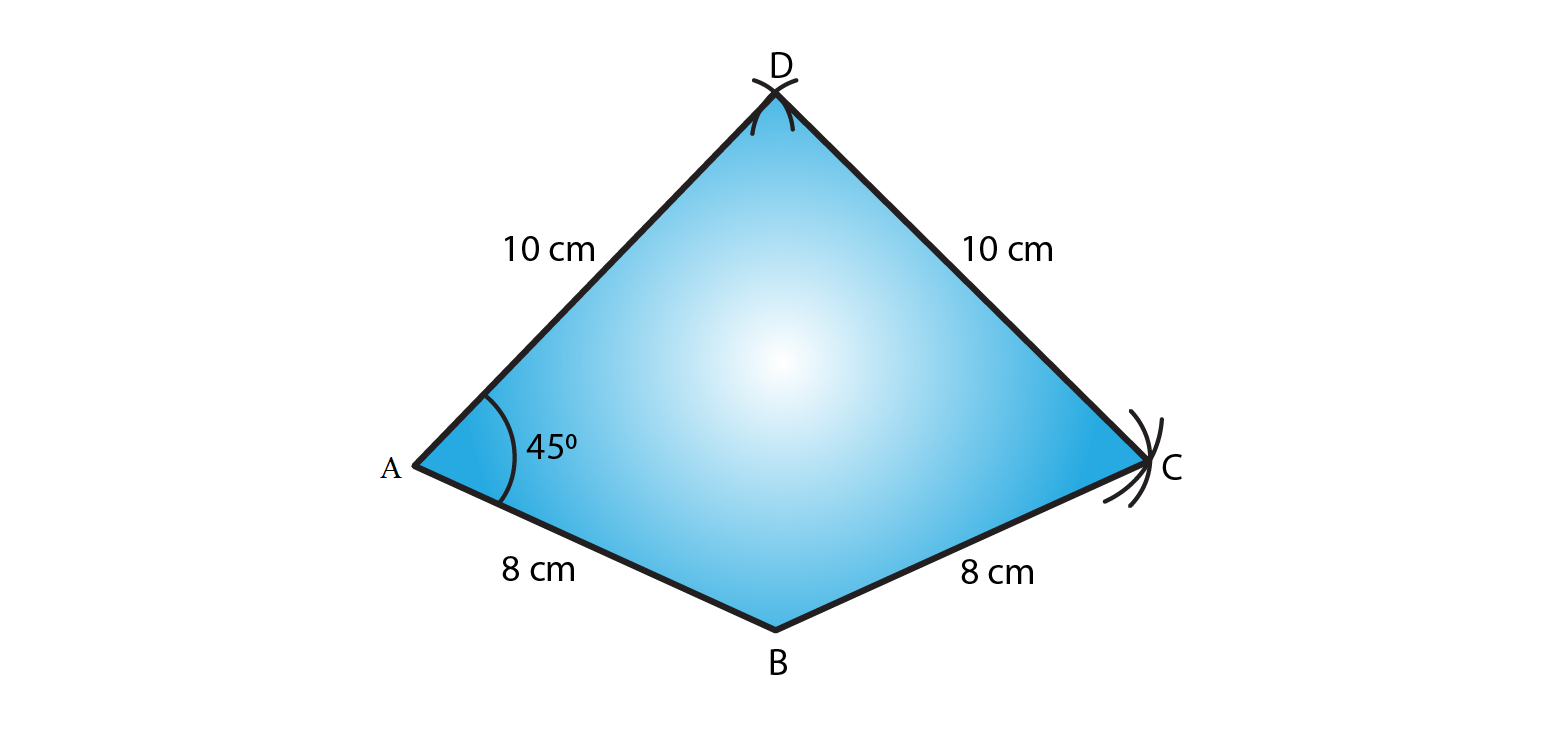3. Construct a quadrilateral ABCD in which AB = 7.7 cm, BC = 6.8 cm, CD = 5.1 cm, AS = 3.6 cm and ∠C = 120°.

Solution:

The given details are AB = 7.7 cm, BC = 6.8 cm, CD = 5.1 cm, AS = 3.6 cm and ∠C = 120°.

Step 1- Draw a line DC = 5.1cm

Step 2- Construct and angle of 120o at C.

Step 3- Cut an arc of radius 6.8cm with C as the center to mark that point as B.

Step 4- Cut an arc of radius 7.7cm with B as the center to mark that point as A.

Step 5- Cut an arc of radius 3.6cm with D as the center to intersect at point A.

Step 6- Now join CB, BA and DA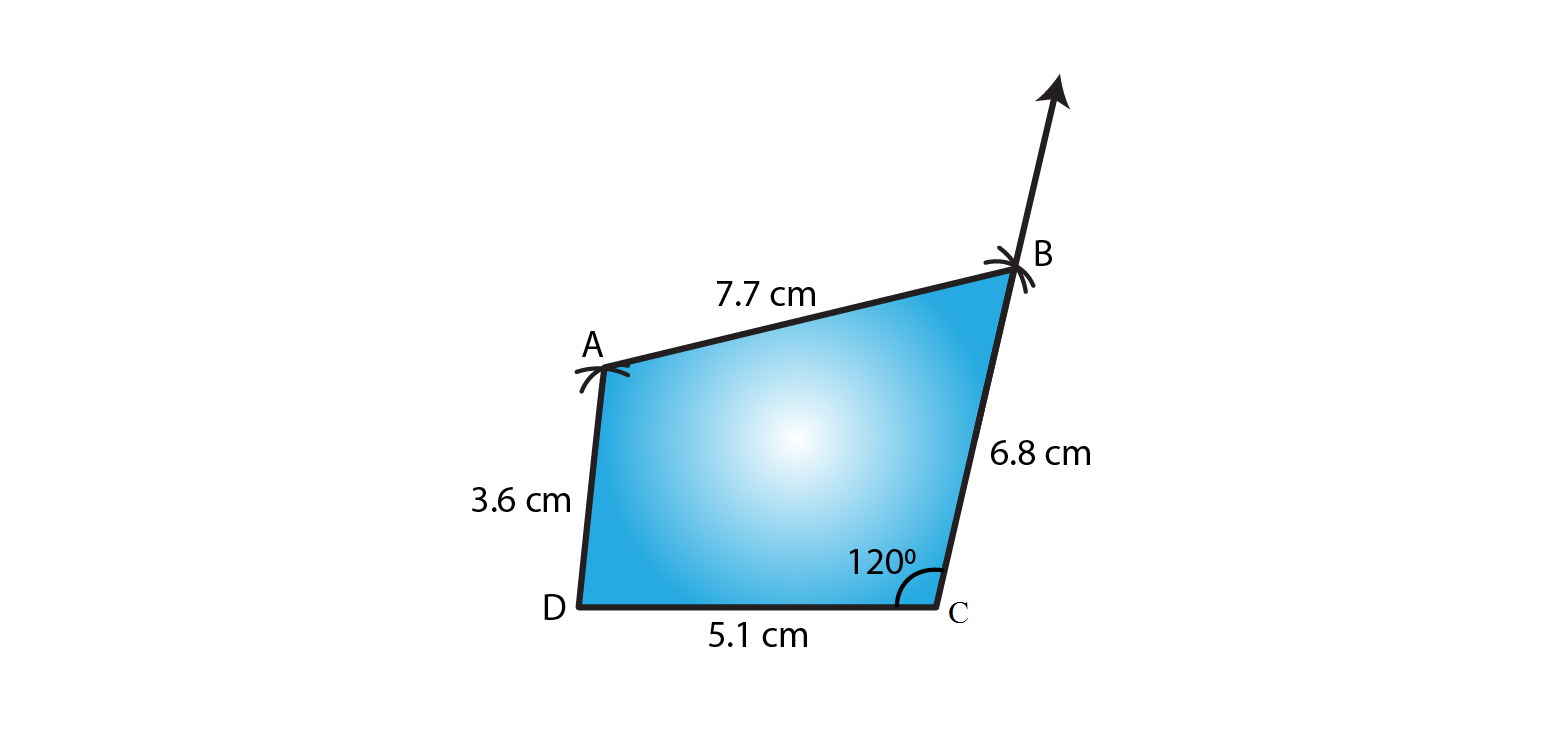4. Construct a quadrilateral ABCD in which AB = BC = 3 cm, AD = CD = 5 cm and ∠B = 120°.

Solution:

The given details are AB = BC = 3 cm, AD = CD = 5 cm and ∠B = 120°.

Step 1- Draw a line AB = 3cm

Step 2- Construct and angle of 120o at B.

Step 3- Cut an arc of radius 3cm with B as the center to mark that point as C.

Step 4- Cut an arc of radius 5cm with C as the center to mark that point as D.

Step 5- Cut an arc of radius 5cm with A as the center to intersect at point D.

Step 6- Now join BC, CD and DA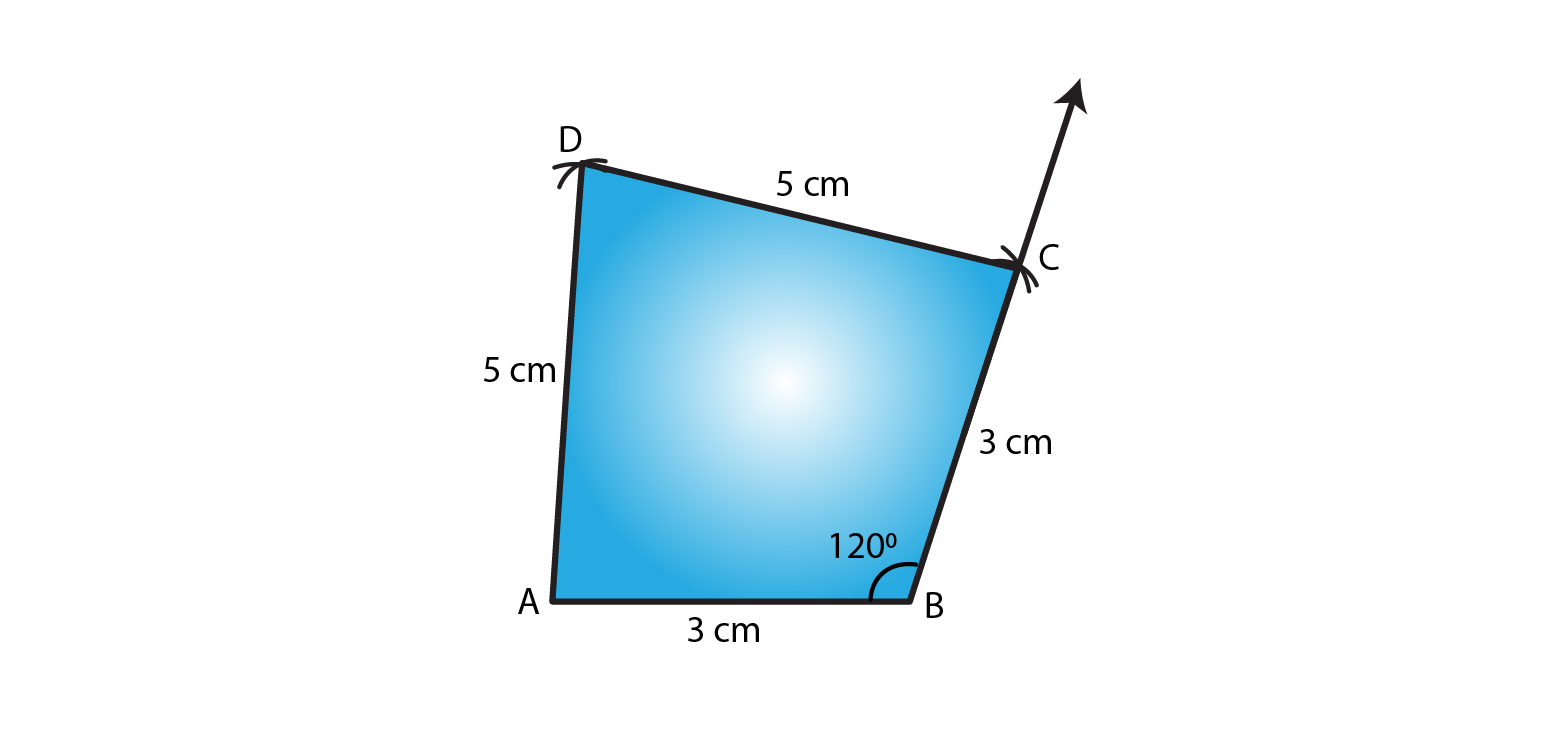5. Construct a quadrilateral ABCD in which AB = 2.8 cm, BC = 3.1 cm, CD = 2.6 cm and DA = 3.3 cm and ∠A = 60°.

Solution:

The given details are AB = 2.8 cm, BC = 3.1 cm, CD = 2.6 cm and DA = 3.3 cm and ∠A = 60°.

Step 1- Draw a line AB = 2.8cm

Step 2- Construct and angle of 60o at A.

Step 3- Cut an arc of radius 3.3cm with A as the center to mark that point as D.

Step 4- Cut an arc of radius 2.6cm with D as the center to mark that point as C.

Step 5- Cut an arc of radius 3.1cm with B as the center to intersect at point C.

Step 6- Now join AD, DC and CB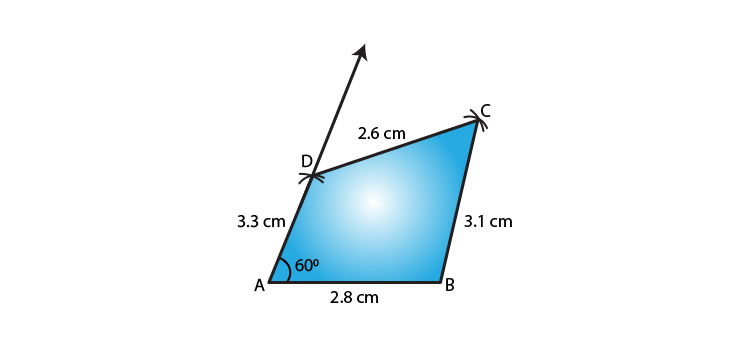6. Construct a quadrilateral ABCD in which AB = BC = 6 cm, AD = DC = 4.5 cm and ∠B = 120°.

Solution:

The given details are AB = BC = 6 cm, AD = DC = 4.5 cm and ∠B = 120°.

Step 1- Draw a line AB = 6cm

Step 2- Construct and angle of 120o at B.

Step 3- Cut an arc of radius 6cm with B as the center to mark that point as C.

Here, AC is about 10.3cm in length which is greater than AD + CD = 4.5+4.5=9cm

We know that sum of the two sides of a triangle is always greater than the third side.